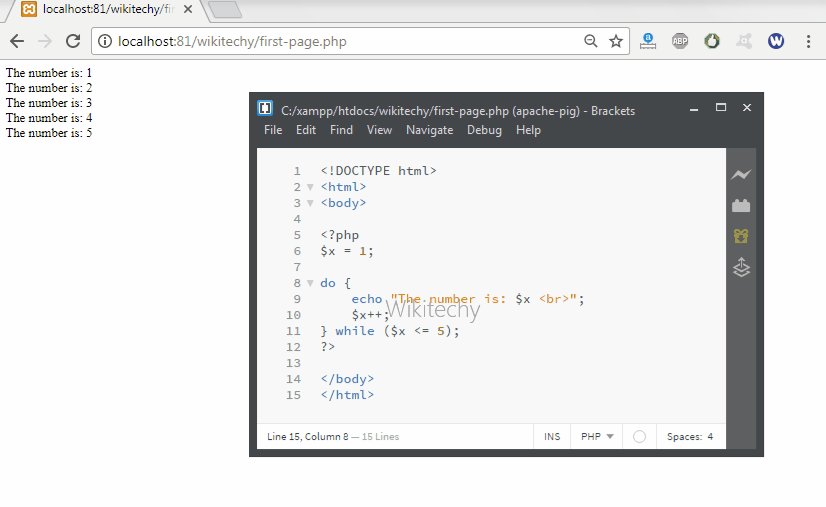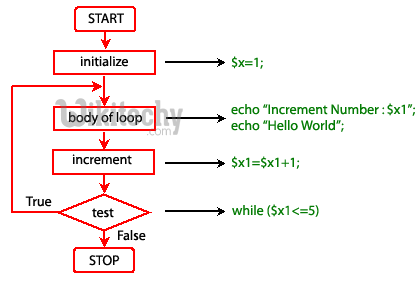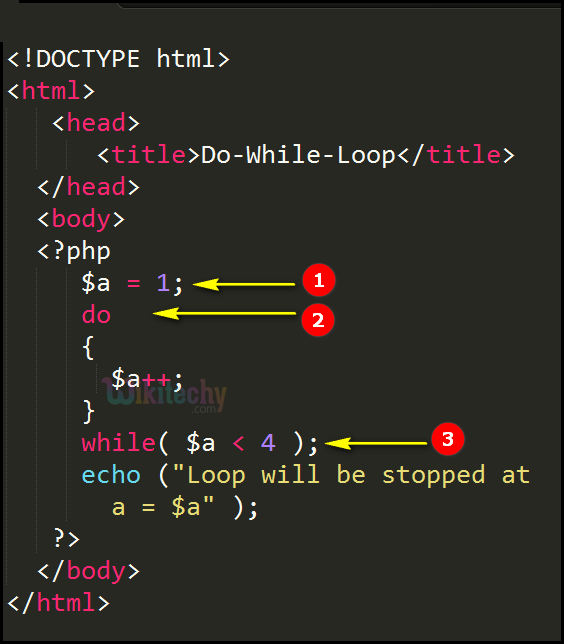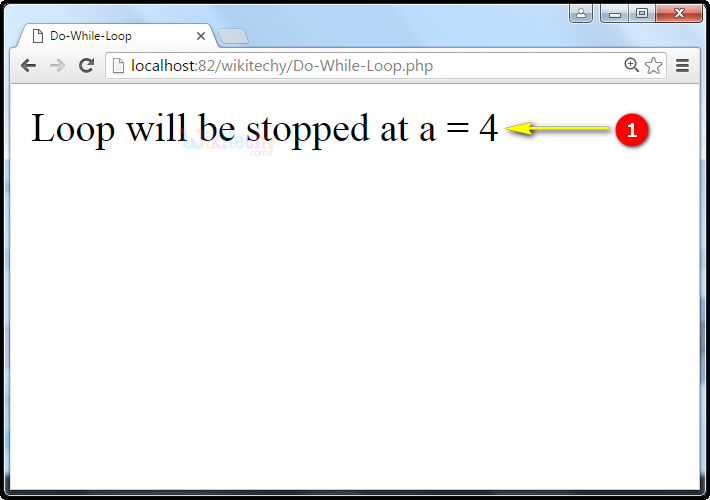# php tutorial - PHP Do While Loop - php programming - learn php - php code - php script

• do-while loops are very similar to while loops, except the truth expression is checked at the end of each iteration instead of in the beginning.
• In PHP do-while is used to provide a control condition.
• In do-while the block of code executed once and then condition is evaluated. If the condition is true, the statement is repeated as long as the specified condition is true.Learn PHP - PHP tutorial - Do While loop - PHP examples - PHP programs

## php programming Syntax :## php for beginners Code Explanation :1. \$a =1 is a variable.
2. In this example do statement specifies the variable \$a which has 1 , that turns out to be true for the condition \$a < 4 i.e.( 1<4 ). Therefore, the loop continues.
3. while(\$a < 4) specifies the condition lesser than 4 which prints the echo statement “Loop will be stopped at a = 4”.

## php tutorials Sample Output :1. Output -> “Loop will be stopped at a = 4“ specifies that the do while loop stopped at 4.